### Quantitative Aptitude Quiz For IBPS PO/Clerk Prelims

Numerical Ability or Quantitative Aptitude Section has given heebie-jeebies to the aspirants when they appear for a banking examination. As the level of every other section is only getting complex and convoluted, there is no doubt that this section, too, makes your blood run cold. The questions asked in this section are calculative and very time-consuming. But once dealt with proper strategy, speed, and accuracy, this section can get you the maximum marks in the examination. Following is the Quantitative Aptitude quiz to help you practice with the best of latest pattern questions.

Q1.if x < y
if x > y
if x ≤ y
if x ≥ y
if x = y or no relation can be established

Q2.if x < y
if x > y
if x ≤ y
if x ≥ y
if x = y or no relation can be established

Q3.if x < y
if x > y
if x ≤ y
if x ≥ y
if x = y or no relation can be established

Q4.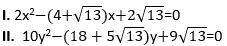if x < y
if x > y
if x ≤ y
if x ≥ y
if x = y or no relation can be established

Q5.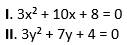if x < y
if x > y
if x ≤ y
if x ≥ y
if x = y or no relation can be established

Q6.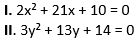if x < y
if x > y
if x ≤ y
if x ≥ y
if x = y or no relation can be established

Q7.if x < y
if x > y
if x ≤ y
if x ≥ y
if x = y or no relation can be established

Q8.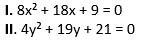if x < y
if x > y
if x ≤ y
if x ≥ y
if x = y or no relation can be established

Q9.if x < y
if x > y
if x ≤ y
if x ≥ y
if x = y or no relation can be established

Q10.if x < y
if x > y
if x ≤ y
if x ≥ y
if x = y or no relation can be established

Q11.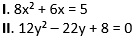if x < y
if x > y
if x ≤ y
if x ≥ y
if x = y or no relation can be established

Q12.if x < y
if x > y
if x ≤ y
if x ≥ y
if x = y or no relation can be established

Q13.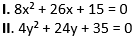if x < y
if x > y
if x ≤ y
if x ≥ y
if x = y or no relation can be established

Q14.if x < y
if x > y
if x ≤ y
if x ≥ y
if x = y or no relation can be established

Q15.if x < y
if x > y
if x ≤ y
if x ≥ y
if x = y or no relation can be established

You May also like to Read: